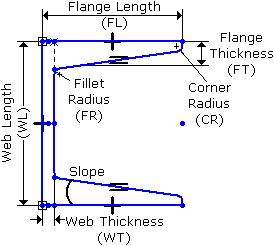# Cross Section Symbol C_SType - Intergraph Smart 3D - Reference Data

## Intergraph Smart 3D Structural Reference Data Overview

PPMProductFamily
3D Design and Visualization
PPMProduct
Intergraph Smart 3D
PPMCategory
Reference Data
Version_S3D
12.x(2018)

The C_SType profile cross section symbol uses the following properties.Section Name

Type the section name. This name displays when you label members. The section name must be unique across the catalog.

Short Name

Type the short name for the section.

Alternate EDI Name

Type the Electronic Data Interchange name for the section. This name is used when translating sections through CIMsteel. This property is currently not used.

Description

Type a short description if needed.

Area

Type the cross-section area for the section. The area is defined in square inches or square millimeters.

Depth

Type the depth for the section (Web Length). The depth is defined in inches or millimeters.

Width

Type the breadth for the section. The breadth is defined in inches or millimeters.

Perimeter

The outside perimeter distance for the section.

CentroidX

Type the location of the centroid along the local x-axis. The location is defined in inches or millimeters.

CentroidY

Type the location of the centroid along the local y-axis. The location is defined in inches or millimeters.

Moment of Inertia about X (Ixx)

Type the moment of inertia for the section's local x- axis.

Moment of Inertia about Y (Iyy)

Type the moment of inertia for the section's local y- axis.

Warping Statical Moment (Sw)

Type the warping statical moment for the section.

Elastic Section Modulus about X (Sxx)

Type the section modulus for the section's local x-axis.

Elastic Section Modulus about Y (Syy)

Type the section modulus for the section's local y-axis.

Torsional Moment of Inertia (J)

Type the torsional moment of inertia for the section.

Warping Constant (Cw)

Type the warping constant for the section.

Flexural Constant (H)

Type the flexural constant for the section.

Polar Radius of Gyration about Shear Center (ro)

Type the polar radius of gyration about the shear center.

Radius of Gyration about X axis (Rxx)

Type the radius of gyration for the section's local x-axis.

Radius of Gyration about Y axis (Ryy)

Type the radius of gyration for the section's local y-axis.

Radius of Gyration about Principle XY (Rxy)

Type the radius of gyration about the local z-axis. The radius is defined in inches or millimeters.

Plastic Section Modulus about X (Zxx)

Type the plastic section modulus for the section's local x-axis.

Plastic Section Modulus about Y (Zyy)

Type the plastic section modulus for the section's local y-axis.

IsHollow

Specify if the cross-section is hollow or not. For this cross-section, the default value is False.

IsSymmetricAlongX

Specify if the cross-section is symmetrical along the local x-axis. For this cross-section, the default value is True.

IsSymmetricAlongY

Specify if the cross-section is symmetrical along the local y-axis. For this cross-section, the default value is False.